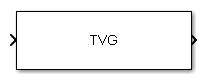# Time Varying Gain

Time varying gain (TVG) control

## Library

Detection

`phaseddetectlib`

•## Description

The Time Varying Gain block applies a time varying gain to input signals to compensate for range loss at each range gate. Time varying gain (TVG) is sometimes called automatic gain control (AGC).

## Parameters

Source of range losses

Specify the range loss source as either `Property` or `Input Port`

 `Property` Range losses are specified by the Range loss (dB) parameter. `Input port` Range losses are specified using the input port `L`.
Range loss (dB)

Specify the loss due to range as a vector — elements correspond to the samples in the input signal. Units are in dB

Reference range loss (dB)

Specify the loss, in dB, at a given reference range as a scalar.

Simulate using

Block simulation method, specified as `Interpreted Execution` or ```Code Generation```. If you want your block to use the MATLAB® interpreter, choose `Interpreted Execution`. If you want your block to run as compiled code, choose `Code Generation`. Compiled code requires time to compile but usually runs faster.

Interpreted execution is useful when you are developing and tuning a model. The block runs the underlying System object™ in MATLAB. You can change and execute your model quickly. When you are satisfied with your results, you can then run the block using ```Code Generation```. Long simulations run faster than they would in interpreted execution. You can run repeated executions without recompiling. However, if you change any block parameters, then the block automatically recompiles before execution.

When setting this parameter, you must take into account the overall model simulation mode. The table shows how the Simulate using parameter interacts with the overall simulation mode.

When the Simulink® model is in `Accelerator` mode, the block mode specified using Simulate using overrides the simulation mode.

Acceleration Modes

 Block Simulation Simulation Behavior `Normal` `Accelerator` `Rapid Accelerator` `Interpreted Execution` The block executes using the MATLAB interpreter. The block executes using the MATLAB interpreter. Creates a standalone executable from the model. `Code Generation` The block is compiled. All blocks in the model are compiled.

## Ports

Note

The block input and output ports correspond to the input and output parameters described in the `step` method of the underlying System object. See link at the bottom of this page.

PortDescriptionSupported Data Types
`In`

Input signal.

The size of the first dimension of the input matrix can vary to simulate a changing signal length. A size change can occur, for example, in the case of a pulse waveform with variable pulse repetition frequency.

Double-precision floating point
`L`

Range loss.

The size of the first dimension of the input matrix can vary to simulate a changing signal length. A size change can occur, for example, in the case of a pulse waveform with variable pulse repetition frequency.

`Out`

Compensated signal.

Double-precision floating point

## Version History

Introduced in R2014b